Starting Time Calculation for Induction Motor | OMICS International
Journal of Electrical & Electronic Systems

# Starting Time Calculation for Induction Motor

Abhishek Garg* and Arun Tomar

L&T Construction, Manapakkam, Chennai, Tamilnadu, India

*Corresponding Author:
Abhishek Garg
Sr. Design Engineer, L&T Construction
Tel: +91-9457047264
E-mail: [email protected]

Received Date: April 06, 2014; Accepted Date: June 27, 2015; Published Date: July 15, 2015

Citation: Garg A, Tomar A (2015) Starting Time Calculation for Induction Motor. J Electr Electron Syst 4:149. doi:10.4172/2332-0796.1000149

Copyright: © 2015 Garg A, et al. This is an open-access article distributed under the terms of the Creative Commons Attribution License, which permits unrestricted use, distribution, and reproduction in any medium, provided the original author and source are credited.

Visit for more related articles at Journal of Electrical & Electronic Systems

#### Abstract

This paper presents the starting time calculation for a squirrel cage induction motor. The importance of starting time lies in determining the duration of large current, which flows during the starting of an induction motor. Normally, the starting current of an induction motor is six to eight time of full load current. Plenty of methods have been discovered to start motor in a quick time, but due to un-economic nature, use are limited. Hence, for large motors direct online starting is most popular amongst all due to its economic and feasible nature. But large current with DOL starting results in a heavy potential drop in the power system. Thus, Special care and attention is required in order to design the healthy system. A very simple method to calculate the starting time of motor is proposed in this paper. Respective simulation study has been carried out using MATLAB 7.8.0 environment, which demonstrates the effectiveness of the starting time calculation.

#### Keywords

Induction motor; Starting time; Direct online starting

#### Introduction

Motors in modern industrial systems are becoming larger due the heavy applications requirement. Some are considered large even in comparison to the total capacity of large industrial power systems. Starting of such large motors can cause adverse effects to any locally connected load, other motors and also to buses, which are electrically remote from the point of motor starting.

Ideally, a motor-starting study should be done prior to purchase of a large motor. The motor manufacturer shall provide the value of starting voltage requirement and preferred locked-rotor current. A motor-starting study should be done if the motor horsepower exceeds approximately 30% of the supply transformer(s) base kVA rating, if no generators are present. Whereas, If generator is present, and no other sources are involved, an analysis should be done whenever the motor horsepower exceeds 10-15% of the generator kVA rating, depending on actual generator characteristics .

Squirrel cage induction motor is most commonly used motor in the world due to its simple design, less maintenance and simple operation. The rating of induction motor is available from fraction of watts to Mega-Watts. It can be used for different type of applications based on torque speed characteristics requirements such as constant power, constant torque, torque increases in proportion to speed, torque increases with the square of speed, torque decreases in inverse proportion to speed .

Many conference papers have been published on motor starting with various starting methods and starting time calculations, but none of them compares the outcome with the simulation software.

This paper presents the comparative study between mathematical calculation and MATLAB result of motor starting time .

#### Basics of Induction Motor

The basic operation of induction motor is similar to transformer, where the stator acts as the primary side of transformer and the rotor as secondary of transformer as shown in Figure 1. At time of starting, the voltage induced in the induction motor rotor is maximum because slip will be maximum (S=1). Since the rotor impedance is low, the rotor current is excessively large. Due to transformer action this large rotor current is reflected in the stator . This results in large starting current (nearly 6 to 8 times the full-load current) in the stator at low power factor and consequently the value of starting torque is low. This large current does not harm the motor due to short duration.

However, this large starting current will produce large drop in linevoltage . Table 1 represents the minimum allowable voltage levels required, when motor starting is taken into consideration.

Voltage drop location or problem Minimum allowable voltage (% rated)
At terminals of starting motor 80%
All terminals of other motors that must reaccelerate 71%
AC contactor pick-up (by standard) (see 9.8, NEMA standards) 85%
DC contactor pick-up (by standard) (see 9.8, NEMA standards) 80%
Contactor hold-in (average of those in use) 60% -70%
Solid-state control devices 90%
Noticeable light flicker 3 % change

Table 1: Summary of representative critical system voltage levels when starting motors (IEEE 399-1997).

The starting torque and starting current also depends on motor class as shown in Table 2. This change is due to change in value of X1 and X2 with different class of motors as shown in Figure 1.

Motor Class Description Fraction of X 1 + X 2
X 1 X 2
A Normal starting torque, normal starting current 0.5 0.5
B Normal starting torque, low starting current 0.4 0.6
C High starting torque, low starting current 0.3 0.7
D High starting torque, high slip 0.5 0.5
Wound rotor Performance varies with rotor resistance 0.5 0.5

Table 2: Empirical distribution of leakage reactance in induction motors (IEEE 112).

So if we are changing X1 and X2, then starting time will also change. If this time is more than limit value, this large current will adversely affect the other electrical equipment connected to the same bus. Figure 2 shows the equivalent circuit of induction motor.

Therefore, it is desirable and necessary to reduce the magnitude of stator current during starting. Several methods have been discovered to reduce the starting current. Some of popular starting methods amongst all are

1) Direct-on-line starting

2) Stator resistance starting

3) Autotransformer starting

4) Star-delta starting

5) Rotor resistance

In practice, any one of the first four methods is used for starting squirrel cage motors, depending upon the size of the motor. But slip ring motors are mostly started by rotor resistance starting.

Stator resistance starting

In stator resistance starting, external resistances are connected in series with each phase of stator winding during starting. This causes voltage drop across the resistances so that voltage available across motor terminals is reduced and hence the starting current. The starting resistances are gradually cut out in steps (two or more steps) from the stator circuit as the motor picks up speed. When the motor attains rated speed, the resistances are completely cut out and full line voltage is applied to the rotor .

This method suffers from two drawbacks. First, the reduced voltage applied to the motor during the starting period lowers the starting torque and hence increases the accelerating time. Secondly, a lot of power is wasted in the starting resistances.

Autotransformer starting

This method also aims at connecting the induction motor to reduced supply at starting and then connecting it to the full voltage as the motor picks up sufficient speed. The tapping on the autotransformer is so set that when it is in the circuit, 65% to 80% of line voltage is applied to the motor. At the instant of starting, the change-over switch is thrown to “start” position. This puts the autotransformer in the circuit and thus reduced voltage is applied to the circuit. Consequently, starting current is limited to safe value. When the motor attains about 80% of normal speed, the changeover switch is thrown to “run” position. This takes out the autotransformer from the circuit and puts the motor to full line voltage . Autotransformer starting has several advantages few of them are low power loss, low starting current and less radiated heat. For large machines (over 25 H.P.), this method of starting is often used. This method can be used for both star and delta connected motors.

Star-delta starting

The stator winding of the motor is designed for delta operation and is connected in star during the starting period. When the machine is up to speed, the connections are changed to delta.

At the instant of starting, the changeover switch is thrown to “Start” position which connects the stator windings in star. Therefore, each stator phase gets volts where V is the line voltage. This reduces the starting current. When the motor picks up speed, the changeover switch is thrown to “Run” position which connects the stator windings in delta. Now each stator phase gets full line voltage V.

The disadvantage of this method is: when there is Star-connection during starting, stator phase voltage is √ times of line voltage. Consequently, starting torque is times the value it would have with D-connection. This method becomes rare for a large motor due to large reduction in starting torque .

#### Importance of Starting Time

Large motor over current protection is normally set to trip prior to the locked-rotor withstand time (LRWT) provided by the motor manufacturer, after the calculated motor start time. The locked-rotor withstand time is determined by the motor designer based on the heating of the rotor parts for locked-rotor condition, where the motor continuously requires a large value of inrush current.

At the time of starting, an induction motor draws high values of current (motor is a constant impedance device during the starting condition), that are very close to the motor’s locked rotor value and remains at this value for the time required to start the motor. This is the reason why the locked-rotor withstand time is used as an allowable time limit for starting the motor across the line, full voltage .

The capability to calculate motor starting time for large induction motor is important in order to evaluate the relative strength of the power system. Typically the motor designer may calculate the motor starting time at a couple of selected values such as 90% or 85% and then plot the results on the time versus current curves for the supplied motor.

System designers can use these starting times, but it is sometimes necessary to calculate the motor starting times using the results of a power system studies for the maximum voltage dip at critical power system conditions. This method can then be used, using the maximum voltage dip, to avoid application problems, set motor protective devices and perform coordination studies with other protective devices on the system.

#### Calculation using Acceleration Torque and Acceleration Time

Figure 3 shows the torque speed curve of induction motor. By this we can see that when we applied voltage on an induction motor it speed increase from zero with the torque. This is clear that at starting slip of induction motor is 1 and with the increase speed there is reduction in slip. Motor come to rated speed near its synchronous speed and the time taken to reach this point is called starting time. By this Figure 3 it is also clear that if gap between the motor torque and load torque is more motors will start very fast and the gap between these two torques is called acceleration torque.

Calculation for acceleration torque and acceleration time is given below with explanation.

Acceleration torque

A load can only be accelerated when the driving motor provides a greater torque than the load requires at the time. The difference of both of these is called the acceleration torque Tα. This acceleration torque will be equal to the multiplication of moment of inertia of motor and angular acceleration of motor. Mathematical formula to calculate this is given in equation 1. Further this angular acceleration can change in term of angular speed and time of starting. In this case the simplified assumption is made that during acceleration period load torque is constant. By calculating average load torques this assumption can be fulfill and replace the variable motor torque by a constant mean acceleration (Figure 4).

Acceleration torque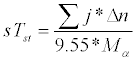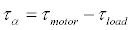(1)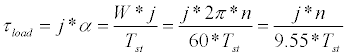(2)

Where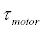= motor torque in Nm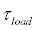= load torque in Nm

Tst = starting time in s

α = angular acceleration /s2

n= motor speed/min

ω = angular speed/s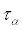= mean acceleration torque in Nm

j = moment of inertia in kg-m2 reduced to the motor shaft

#### Acceleration Time

The acceleration time (starting time) tst of induction motor can be determined from equation 2 by doing some manipulations. As told above that time is time taken by induction motor to reach at rated speed and rated torque at rated voltage. Now if the mean acceleration torque Tα and moment of inertia is known we can easily calculate the value of starting time.

Starting time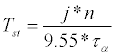(3)

One example has been shown below to understand the concept carefully.

Example: Let an eight-pole SCIM motor with Nr=741 rpm, P=400 kW have an inertia of J=30 kgm2 at no-load and have an average acceleration torque τα =1.5 τmotor (at no load), the maximum time specified by the manufacturer is 15 sec.

Calculate

a) The starting time at no-load?

b) The starting time together with driven equipment torque with reference to motor is 19 Kgm2.

Solution:

a)

Rated torque of the motor

#### Discussion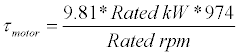So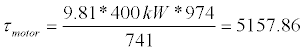(4)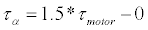As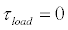So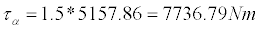Now by Equation (2)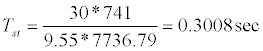(b) Acceleration (starting) time with load Rated torque of the motor

So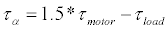But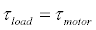at load (5)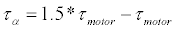So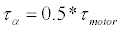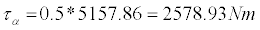At load total moment of inertia in kg-m2=30+19=49kg-m2

Now by Equation (2)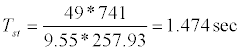In this motor the acceleration time Tst is lesser than the maximum time specified by the manufacturer. Unloaded motors and motors with only little additional centrifugal masses reach their idle speed very quickly. But when large centrifugal masses are to be accelerated, starting times are generally quite high. This is called heavy starting, which is the case, for example, in centrifuges, ball mills, transport systems and large fans. These applications often require special motors and corresponding switch gears.

If the curve of the load torque τload is complex and the motor torque τmotor is not constant, it is advantageous to divide the computation into individual zones as shown in Figure 5.

Now the acceleration times for the individual zones plus the average acceleration torques which take effect in the segment are computed and added up for each and every individual speed segments.

Now to calculate the starting time of non-constant acceleration torque is given by equation 6.

Acceleration time for non-constant torques

in(6)

Where the meaning of each is same as in equation 2, except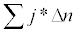This shows sum all zones with multiplication of moment of inertia and change in speed.

#### Simulation Result

Simulation study is carried out by using MATLAB 7.8.0 software with the same eight-pole SCIM motor with Nr=741 rpm, P=400 kW as considered in the previous example. Figure 5 shows that there is nearly six time of rated current during motor starting which is gradually decreasing and reaches to the rated current within 0.7 sec. This time is nearly equal to the time calculated by equation 5. Figure 6 shows the speed vs. time curve obtained by simulation it is clear by the graph that speed is increasing with time and coming to rated value in approximately 0.7 seconds, which is in line with the result calculated by the mathematical equation.

#### Conclusion

In this paper the importance of induction motor starting time has been described along-with different type of starting methods. A mathematical formula to calculate the starting time using acceleration torque, motor speed and moment of inertia of an induction motor is presented and the derived results from the calculation has been verified against the simulation study carried on MATLAB environment. Results of both the approaches are found to be in line with each other.

#### References

Select your language of interest to view the total content in your interested language

### Article Usage

• Total views: 56085
• [From(publication date):
September-2015 - Dec 16, 2019]
• Breakdown by view type
• HTML page views : 50180Can't read the image? click here to refresh